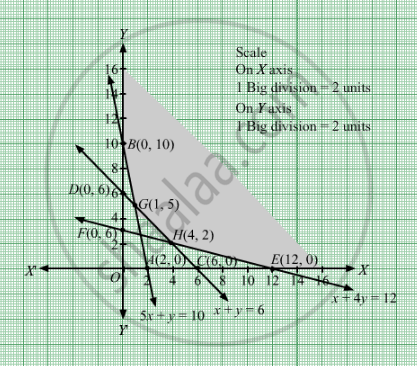# Div » P Show the Solution Zone of the Following Inequalities on a Graph Paper: 5 X + Y ≥ 10 X + Y ≥ 6 X + 4 Y ≥ 12 X ≥ 0 , Y ≥ 0 - Mathematics

Show the solution zone of the following inequalities on a graph paper:

$5x + y \geq 10$

$x + y \geq 6$

$x + 4y \geq 12$

$x \geq 0, y \geq 0$

Find x and y for which 3x + 2y is minimum subject to these inequalities. Use a graphical method.

#### Solution

First, we will convert the given inequations into equations, we obtain the following equations:
5x y = 10, x +y = 6, x + 4y = 12, x = 0 and y = 0
Region represented by 5x ≥ 10:
The line 5x y = 10  meets the coordinate axes at A(2, 0) and B(0, 10) respectively. By joining these points we obtain the line 5x y = 10.
Clearly (0,0) does not satisfies the inequation 5x ≥ 10. So,the region in xy plane which does not contain the origin represents the solution set of the inequation 5x ≥ 10.
Region represented by x +y  ≥ 6:
The line x +y = 6 meets the coordinate axes at C(6,0) and D(0, 6) respectively. By joining these points we obtain the line
2x +3y = 30.Clearly (0,0) does not satisfies the inequation x +y  ≥ 6. So,the region which does not contain the origin represents the solution set of the inequation 2x+3y ≥ 30.
Region represented by x + 4y ≥ 12
The line x + 4y = 12 meets the coordinate axes at E(12, 0) and F(0, 3) respectively. By joining these points we obtain the line
x + 4y = 12.Clearly (0,0) does not satisfies the inequation x + 4y ≥ 12. So,the region which does not contain the origin represents the solution set of the inequation x + 4y≥  12.
Region represented by x ≥ 0 and y ≥ 0:
Since, every point in the first quadrant satisfies these inequations. So, the first quadrant is the region represented by the inequations x ≥ 0, and ≥ 0.
The feasible region determined by the system of constraints 5x ≥ 10, x +y  ≥ 6,x + 4y ≥ 12, x ≥ 0, and y ≥ 0, are as follows ..

The corner points of the feasible region are B(0, 10), G(1,5), H(4,2) and E(12,0).

The values of Z at these corner points are as follows.

 Corner point Z = 3x + 2y B(0, 10) 3 × 0 + 3 × 10 = 30 G(1,5) 3 × 1 + 2 × 5 = 13 H(4,2) 3 × 4 + 2 × 2 = 16 E(12,0) 3 × 12 + 2 × 0 = 36

Therefore, the minimum value of Z is 13 at the point G(1,5). Hence, x = 1 and y = 5 is the optimal solution of the given LPP.
Thus, the optimal value of Z is 13.

Concept: Graphical Method of Solving Linear Programming Problems
Is there an error in this question or solution?

#### APPEARS IN

RD Sharma Class 12 Maths
Chapter 30 Linear programming
Exercise 30.2 | Q 22 | Page 33